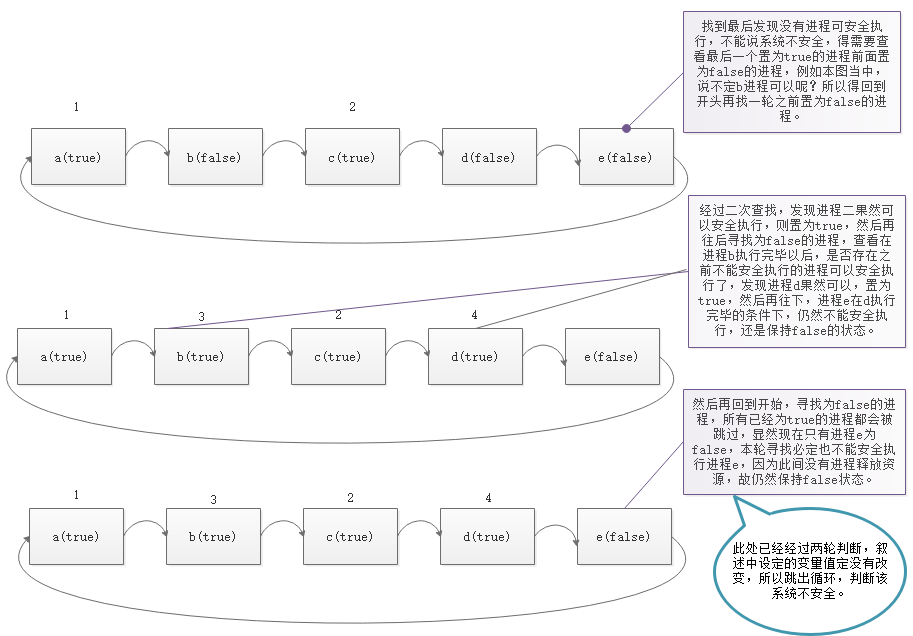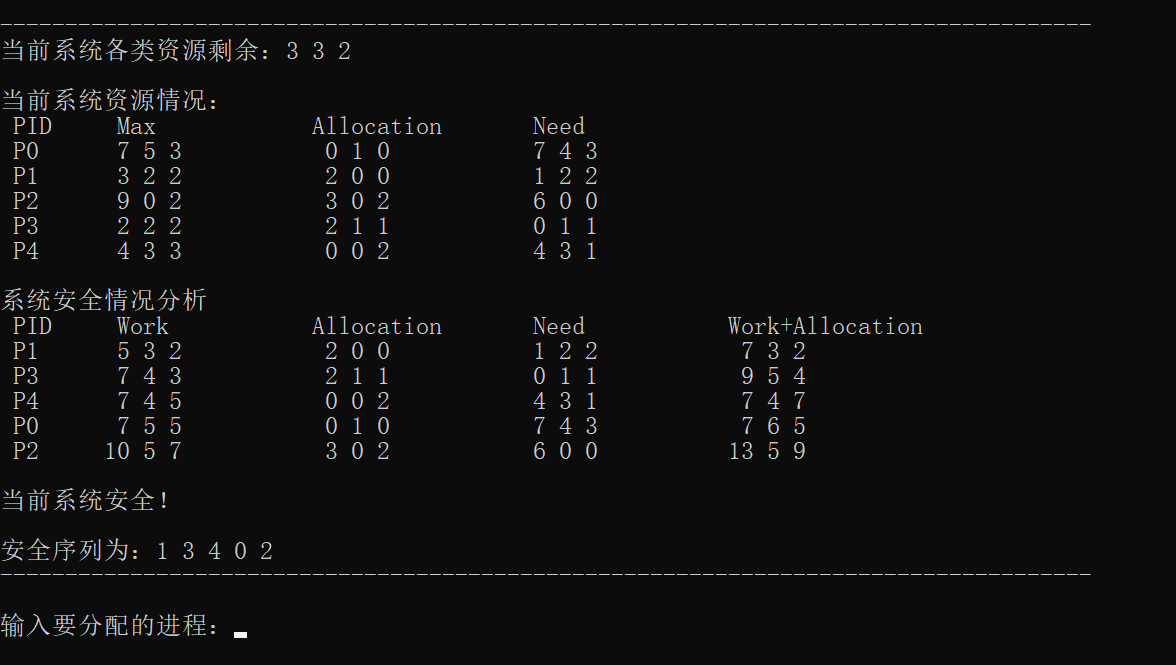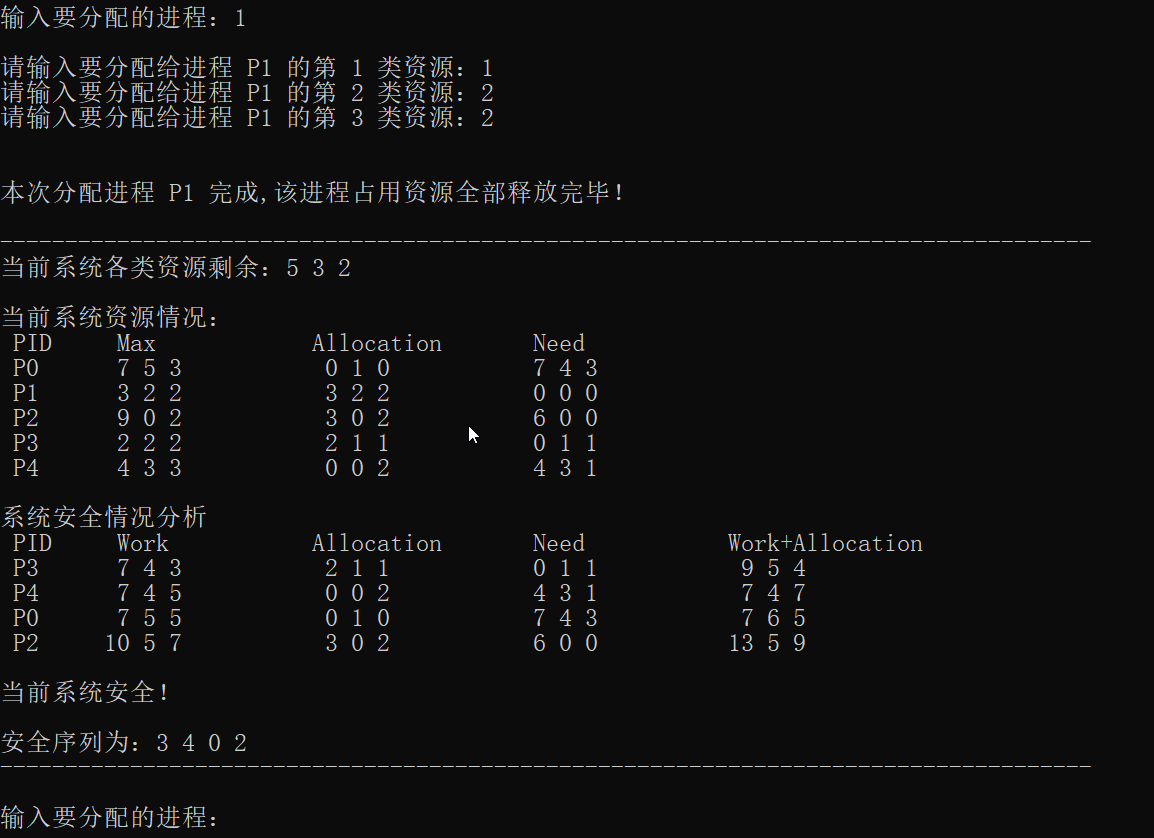Banker's Algorithm

•JS
• ## 计算机操作系统_银行家算法

万次阅读 多人点赞 2018-12-05 23:21:02
银行家算法
银行家算法
什么是银行家算法?
银行家算法（Banker’s Algorithm）是一个避免死锁（Deadlock）的著名算法，是由艾兹格·迪杰斯特拉在1965年为T.H.E系统设计的一种避免死锁产生的算法。它以银行借贷系统的分配策略为基础，判断并保证系统的安全运行。
在银行中，客户申请贷款的数量是有限的，每个客户在第一次申请贷款时要声明完成该项目所需的最大资金量，在满足所有贷款要求时，客户应及时归还。银行家在客户申请的贷款数量不超过自己拥有的最大值时，都应尽量满足客户的需要。在这样的描述中，银行家就好比操作系统，资金就是资源，客户就相当于要申请资源的进程。
银行家算法是一种最有代表性的避免死锁的算法。在避免死锁方法中允许进程动态地申请资源，但系统在进行资源分配之前，应先计算此次分配资源的安全性，若分配不会导致系统进入不安全状态，则分配，否则等待。为实现银行家算法，系统必须设置若干数据结构。

银行家算法中的数据结构
为了实现银行家算法，在系统中必须设置这样四个数据结构，分别用来描述系统中可利用的资源、所有进程对资源的最大需求、系统中的资源分配，以及所有进程还需要多少资源的情况。
(1)  可利用资源向量 Available。这是一个含有 m 个元素的数组，其中的每一个元素代表一类可利用的资源数目，其初始值是系统中所配置的该类全部可用资源的数目，其数值随该类资源的分配和回收而动态地改变。如果 Available[j] = K，则表示系统中现Rj类资源K个。
(2)  最大需求矩阵Max。这是一个n x m的矩阵，它定义了系统中n个进程中的每个进程对m类资源的最大需求。如果Max[i,j] = K，则表示进程i需要Rj 类资源的最大数目为K。
(3)  分配矩阵 Allocation。这也是一个n x m的矩阵，它定义了系统中每一类资源当前已分配给每一进程的资源数。如果 Allocation[i,jl = K，则表示进程i当前己分得Rj类资源的数目为K。
(4)  需求矩阵Need.这也是一个n×m的矩阵，用以表示每一个进程尚需的各类资源数。如果Need[i,j] = K，则表示进程i还需要Rj类资源K个方能完成其任务。
上述三个矩阵间存在下述关系:
Need[i,j] = Max[i,j] - allocation[i, j]

银行家算法详述：
设 Request；是进程Pi的请求向量，如果 Requesti[j] = K，表示进程Pi需要K个Rj类型的资源。当Pi发出资源请求后，系统按下述步骤进行检査:
(1)  如果 Requesti[j] ≤ Need[i,j]便转向步骤(2)；否则认为出错，因为它所需要的资源数已超过它所宣布的最大值。
(2)  如果 Requesti[j] ≤ Available[j]，便转向步骤(3)；否则，表示尚无足够资源，Pi须等待。
(3)  系统试探着把资源分配给进程Pi，并修改下面数据结构中的数值
Available[j] = Available[j] - Requesti[j];
Allocation[i,j] = Allocation[i,j] + Requesti[j];
Need[i,j] = Need[i,j] - Requesti[j];
(4)  系统执行安全性算法，检查此次资源分配后系统是否处于安全状态。若安全，才正式将资源分配给进程Pi，以完成本次分配；否则，将本次的试探分配作废，恢复原来的资源分配状态，让进程Pi等待。

安全性算法:
系统所执行的安全性算法可描述如下:
(1)  设置两个向量:①工作向量Work，它表示系统可提供给进程继续运行所需的各类资源数目，它含有m个元素，在执行安全算法开始时，Work = Available；② Finish:它表示系统是否有足够的资源分配给进程，使之运行完成。开始时先做 Finish[i] = false；当有足够资源分配给进程时，再令Finish[i] = true。
(2)  从进程集合中找到一个能满足下述条件的进程
① Finish[i] = false;
② Need[i,j] ≤ Work[j];
若找到，执行步骤(3)，否则，执行步骤(4)。
(3)当进程Pi获得资源后，可顺利执行，直至完成，并释放出分配给它的资源，故应执行:
Work[j] = Work[j] + Allocation[i,j];
Finish[i] = true;
go to step 2;(goto语句不推荐使用 _ )
(4)如果所有进程的 Finish[i] =true都满足，则表示系统处于安全状态；否则，系统处于不安全状态。

难点透析：
本程序的难点在于安全性算法，对于一个安全的系统来说，此步骤较为容易，难在于判断不安全的系统。为什么这么说呢？由于本程序再设计寻找安全序列的部分使用while循环，就需要找到分别处理安全系统与不安全系统的终止循环条件，对于安全的系统，满足条件 Finish[i] = false 和  Need[i,j] ≤ Work[j] 的，必定也会按照预期的将 Finish[i] 向量全部置为true，那是不是就可以设置一个变量来累加计数，当该变量与进程数量相等的时候，就说明已经全部置为true了，终止循环，也就是说系统安全。
对于不安全的系统，上述方法肯定是不行的，因为不可能将向量 Finish[i] 都置为 true ，必定存在 false。就得寻求一个跳出循环的条件，但是由于需要不断循环查找并尝试分配，寻求一个安全序列，到底该怎么算是已经找不到安全路径了呢？下面说本程序的解决办法，首先需要想到的是，当我们寻找一轮都没有找到一个可以安全执行的进程，是不是就说明往后也找不到了呢？没错，就是这样的！所以我们每次在记录 Finish[i] = true 的次数的时候，不妨把这个次数再使用另一个变量存放起来，然后在判断语句当中判断当寻找一轮下来，该值未发生改变，说明已经找不到安全的进程了，即可跳出循环，该系统不安全！
图示：部分效果图：完整代码：
#include<stdio.h>
#define resourceNum 3
#define processNum  5

//系统可用（剩余）资源
int available[resourceNum]={3,3,2};
//进程的最大需求
int maxRequest[processNum][resourceNum]={{7,5,3},{3,2,2},{9,0,2},{2,2,2},{4,3,3}};
//进程已经占有（分配）资源
int allocation[processNum][resourceNum]={{0,1,0},{2,0,0},{3,0,2},{2,1,1},{0,0,2}};
//进程还需要资源
int need[processNum][resourceNum]={{7,4,3},{1,2,2},{6,0,0},{0,1,1},{4,3,1}};
//是否安全
bool Finish[processNum];
//安全序列号
int safeSeries[processNum]={0,0,0,0,0};
//进程请求资源量
int request[resourceNum];
//资源数量计数
int num;

//打印输出系统信息
void showInfo()
{
printf("\n------------------------------------------------------------------------------------\n");
printf("当前系统各类资源剩余：");
for(int j = 0; j < resourceNum; j++)
{
printf("%d ",available[j]);
}
printf("\n\n当前系统资源情况：\n");
printf(" PID\t Max\t\tAllocation\t Need\n");
for(int i = 0; i < processNum; i++)
{
printf(" P%d\t",i);
for(int j = 0; j < resourceNum; j++)
{
printf("%2d",maxRequest[i][j]);
}
printf("\t\t");
for(j = 0; j < resourceNum; j++)
{
printf("%2d",allocation[i][j]);
}
printf("\t\t");
for(j = 0; j < resourceNum; j++)
{
printf("%2d",need[i][j]);
}
printf("\n");
}
}

//打印安全检查信息
void SafeInfo(int *work, int i)
{
int j;
printf(" P%d\t",i);
for(j = 0; j < resourceNum; j++)
{
printf("%2d",work[j]);
}
printf("\t\t");
for(j = 0; j < resourceNum; j++)
{
printf("%2d",allocation[i][j]);
}
printf("\t\t");
for(j = 0; j < resourceNum; j++)
{
printf("%2d",need[i][j]);
}
printf("\t\t");
for(j = 0; j < resourceNum; j++)
{
printf("%2d",allocation[i][j]+work[j]);
}
printf("\n");
}

//判断一个进程的资源是否全为零
bool isAllZero(int kang)
{
num = 0;
for(int i = 0; i < resourceNum; i++ )
{
if(need[kang][i] == 0)
{
num ++;
}
}
if(num == resourceNum)
{
return true;
}
else
{
return false;
}
}

//安全检查
bool isSafe()
{
//int resourceNumFinish = 0;
int safeIndex = 0;
int allFinish = 0;
int work[resourceNum] = {0};
int r = 0;
int temp = 0;
int pNum = 0;
//预分配为了保护available[]
for(int i = 0; i < resourceNum; i++)
{
work[i] = available[i];
}
//把未完成进程置为false
for(i = 0; i < processNum; i++)
{
bool result = isAllZero(i);
if(result == true)
{
Finish[i] = true;
allFinish++;
}
else
{
Finish[i] = false;
}

}
//预分配开始
while(allFinish != processNum)
{
num = 0;
for(i = 0; i < resourceNum; i++)
{
if(need[r][i] <= work[i] && Finish[r] == false)
{
num ++;
}
}
if(num == resourceNum)
{
for(i = 0; i < resourceNum; i++ )
{
work[i] = work[i] + allocation[r][i];
}
allFinish ++;
SafeInfo(work,r);
safeSeries[safeIndex] = r;
safeIndex ++;
Finish[r] = true;
}
r ++;//该式必须在此处
if(r >= processNum)
{
r = r % processNum;
if(temp == allFinish)
{
break;
}
temp = allFinish;
}
pNum = allFinish;
}
//判断系统是否安全
for(i = 0; i < processNum; i++)
{
if(Finish[i] == false)
{
printf("\n当前系统不安全！\n\n");
return false;
}
}
//打印安全序列
printf("\n当前系统安全！\n\n安全序列为：");
for(i = 0; i < processNum; i++)
{
bool result = isAllZero(i);
if(result == true)
{
pNum --;
}
}
for(i = 0; i < pNum; i++)
{
printf("%d ",safeSeries[i]);
}
return true;
}

//主函数
void main()
{
int curProcess = 0;
int a = -1;
showInfo();
printf("\n系统安全情况分析\n");
printf(" PID\t Work\t\tAllocation\t Need\t\tWork+Allocation\n");
bool isStart = isSafe();
//用户输入或者预设系统资源分配合理才能继续进行进程分配工作
while(isStart)
{
//限制用户输入，以防用户输入大于进程数量的数字，以及输入其他字符（乱输是不允许的）
do
{
if(curProcess >= processNum || a == 0)
{
printf("\n请不要输入超出进程数量的值或者其他字符：\n");
while(getchar() != '\n'){};//清空缓冲区
a = -1;
}
printf("\n------------------------------------------------------------------------------------\n");
printf("\n输入要分配的进程：");
a = scanf("%d",&curProcess);
printf("\n");

}while(curProcess >= processNum || a == 0);

//限制用户输入，此处只接受数字，以防用户输入其他字符（乱输是不允许的）
for(int i = 0; i < resourceNum; i++)
{
do
{
if(a == 0)
{
printf("\n请不要输入除数字以外的其他字符，请重新输入：\n");
while(getchar() != '\n'){};//清空缓冲区
a = -1;
}
printf("请输入要分配给进程 P%d 的第 %d 类资源：",curProcess,i+1);
a = scanf("%d", &request[i]);
}while( a == 0);
}

//判断用户输入的分配是否合理，如果合理，开始进行预分配
num  = 0;
for(i = 0; i < resourceNum; i++)
{
if(request[i] <= need[curProcess][i] && request[i] <= available[i])
{
num ++;
}
else
{
printf("\n发生错误！可能原因如下：\n(1)您请求分配的资源可能大于该进程的某些资源的最大需要！\n(2)系统所剩的资源已经不足了！\n");
break;
}
}
if(num == resourceNum)
{
num = 0;
for(int j = 0; j < resourceNum; j++)
{
//分配资源
available[j] = available[j] - request[j];
allocation[curProcess][j] = allocation[curProcess][j] + request[j];
need[curProcess][j] = need[curProcess][j] - request[j];
//记录分配以后，是否该进程需要值为0了
if(need[curProcess][j] == 0)
{
num ++;
}
}
//如果分配以后出现该进程对所有资源的需求为0了，即刻释放该进程占用资源（视为完成）
if(num == resourceNum)
{
//释放已完成资源
for(int i = 0; i < resourceNum; i++ )
{
available[i] = available[i] + allocation[curProcess][i];
}
printf("\n\n本次分配进程 P%d 完成,该进程占用资源全部释放完毕！\n",curProcess);
}
else
{
//资源分配可以不用一次性满足进程需求
printf("\n\n本次分配进程 P%d 未完成！\n",curProcess);
}

showInfo();
printf("\n系统安全情况分析\n");
printf(" PID\t Work\t\tAllocation\t Need\t\tWork+Allocation\n");

//预分配完成以后，判断该系统是否安全，若安全，则可继续进行分配，若不安全，将已经分配的资源换回来
if(!isSafe())
{
for(int j = 0; j < resourceNum; j++)
{
available[j] = available[j] + request[j];
allocation[curProcess][j] = allocation[curProcess][j] - request[j];
need[curProcess][j] = need[curProcess][j] +request[j];
}
printf("资源不足，等待中...\n\n分配失败！\n");
}
}
}
}

参考文献：计算机操作系统/汤小丹等编著.-4版.-西安:西安电子科技大学出版社，2014.5(2016.4重印)
如有错误，欢迎指正！


展开全文...